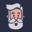Related Tags

javascript
community creator

# How to do basic math operations in JavaScriptCoderslang Master

JavaScript supports all basic arithmetic operations. In this guide, we’ll dive into them and see how the regular +, -, *, and / perform on different data types.

You can use the binary operator + to add two numbers in JS. To operator is binary because it needs exactly two operands (numbers in our case) to perform the desired action.

const sum = 2 + 2;
console.log(sum)

So, the result is exactly what you would expect here, but things will change when we start using other data types. For example, if one of the operands is a string, then the other one will also be considered a string. These two strings will be concatenated"glued" together.

const numberPlusString = 2 + '2';
const booleanPlusString = true + 'Story';
const stringPlusString = 'just' + 'Strings';

console.log(numberPlusString);
console.log(booleanPlusString);
console.log(stringPlusString);

You can also place a regular object, an array, or a function on any side of the + operator. In this case, they will first be converted to a string and then the addition will be done.

const f = () => {return 0};
const obj = {type: 'regular'};
const arr = [1, 2, 3];

console.log('Hello!' + f);
console.log(true + obj);
console.log(1 + arr); 

Notice that most objects will be converted to strings as [object Object]. If you want to do something different, then you should implement a custom toString() function.

const obj = {
type: 'regular',
toString: function () {
return JSON.stringify(this);
},
};

console.log(1 + obj);

Interesting things happen when both operands are boolean or when one of them is a boolean and another one is a number. In this case, true will always be converted to 1 and false will become 0.

const truePlusTrue = true + true;
const truePlusFalse = true + false;
const booleanPlusNumber = true + 5;

console.log(truePlusTrue);
console.log(truePlusFalse);
console.log(booleanPlusNumber);

## Subtraction, multiplication, and division

Even though the rules for addition might seem quite complex, other basic operations follow common logic. With numbers, everything is as expected.

const subtractionResult = 10 - 2;
const multiplicationResult = 2 * 2;
const divisionResult = 10 / 2;

console.log(subtractionResult);
console.log(multiplicationResult);
console.log(divisionResult);

Booleans are still converted to either 0 or 1 when on the other side is a boolean or a number.

console.log(true / true);
console.log(5 * false);
console.log(true - false);

## Infinity and -Infinity

If you try to divide something by 0 or false, then the result is either Infinity or -Infinity.

console.log(5 / 0);
console.log(-5 / false);

## NaN

In most other cases, when it’s hard to make sense of the arithmetic expression, the result will be NaN or “not-a-number”.

console.log(false / false);                     // NaN
console.log(10 / 'string');                     // NaN
console.log(5 * {});                            // NaN
console.log({} - [])                            // NaN

## Empty Array

An empty array is converted into a 0 or an empty string whenever possible.

console.log('str1' + [] + 'str2');               // str1str2
console.log(12 * []);                            // 0
console.log(5 - []);                             // 5
console.log(1 / []);                             // Infinity

## Unary increment and decrement

Two very useful operators allow you to either increment or decrement the value of the variable by 1. They look like double plus (++) and double minus (--).

console.log('str1' + [] + 'str2');               // str1str2
console.log(12 * []);                            // 0
console.log(5 - []);                             // 5
console.log(1 / []);                             // Infinity

The ++ and -- operators can be placed on either side of the variable. Both counter++ and ++counter expressions are valid. The difference can be represented by these examples:

let i = 0;
console.log(i++);                                 // 0
console.log(i);                                   // 1

First, we took the value of i, logged it into the screen, and did the increment. We will see this increment in the second console.log.

With ++i it’s the other way around.

let i = 0;
console.log(++i);                                  // 1
console.log(i);                                    // 1

let x = 1;
let y = 2;
let z = 3;

console.log(++x - y-- + z++);                       // ?

## Conclusion

We’ve covered the basic arithmetic operations in JavaScript, their rules, and exceptions.

The +, -, *, / behave as expected with numbers, but with strings, objects, arrays, functions, and booleans it changes a lot.

Learning the fundamentals of JS (like math) is useful for any web developer.

I write a programming blog at learn.coderslang.com and teach Full Stack JavaScript programming.

Talk soon!

RELATED TAGS

javascript
community creator

CONTRIBUTORCoderslang Master
RELATED COURSES

View all Courses

Keep Exploring

Learn in-demand tech skills in half the time